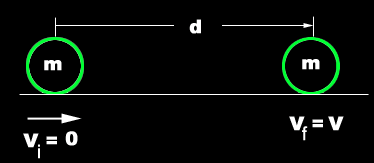# Kinetic Energy

Definition
“Energy posses by a body by virtue of its motion is referred to as ‘Kinetic Energy’”.

Formula
K.E. = 1/2 mv2
Kinetic energy depends upon the mass and velocity of body.
If velocity is zero than K.E. of body will also be zero.
Kinetic energy is a scalar quantity like other forms of energies.

Derive: K.E = 1/2 mv2
Proof
Consider a body of mass “m” starts moving from rest. After a time interval “t” its velocity becomes V.
If initial velocity of the body is Vi = 0 ,final velocity Vf = V and the displacement of body is “d”. ThenFirst we will find the acceleration of body.
Using equation of motion
2aS = Vf2 – Vi2
Putting the above mentioned values
a = V2/2d
Now force is given by
F = ma
Putting the value of acceleration
F = m(V2/2d)
As we know that
Work done = Fd
Putting the value of F
Work done = (mv2/2d)(d)
Work done = mV2/2
OR
Work done = ½ mV2
Since the work done is motion is called “Kinetic Energy”
i.e.
K.E. = Work done
OR
K.E. =1/2mV2.